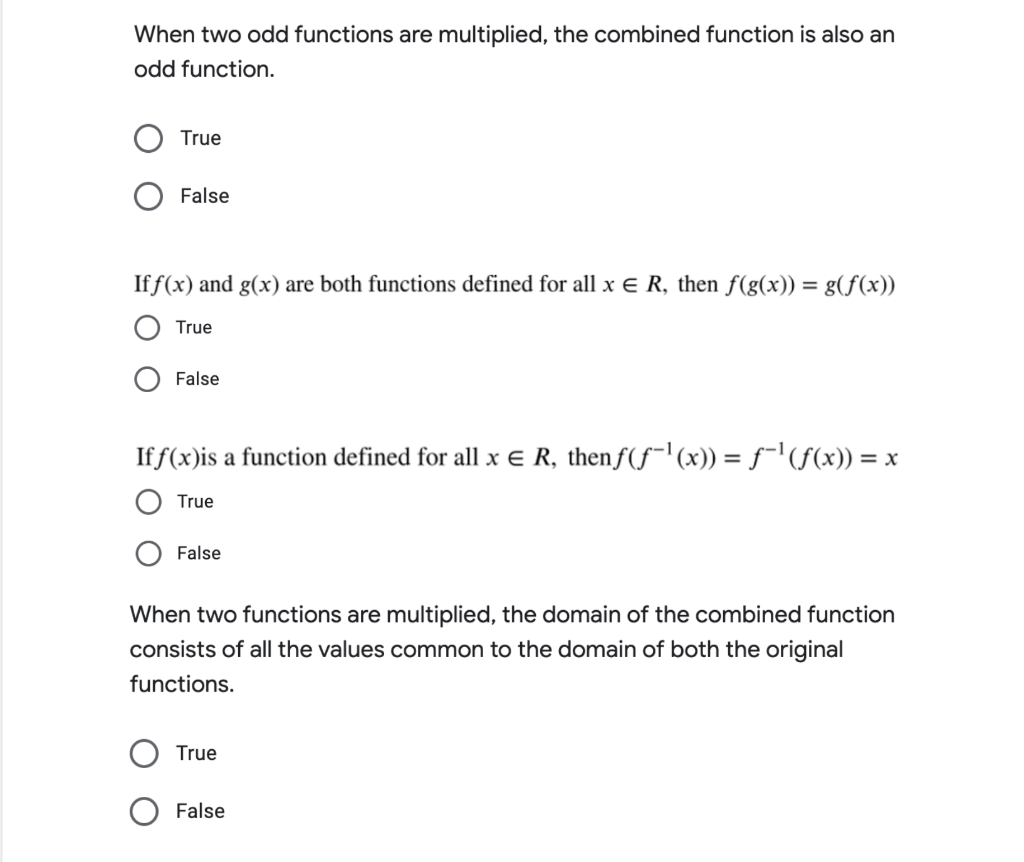# Question Help!] When two odd functions are multiplied, the combined function is also an odd function. True False If f(x) and g(x) are both functions defined for all x € R, then f(g(x)) = g(f(x)) True False If f(x)is a function defined for all x € R, thenƒ(ƒ¯¹(x)) = ƒ¯¹ (ƒ(x)) = x True False When two functions are multiplied, the domain of the combined function consists of all the values common to the domain of both the original functions. True False]